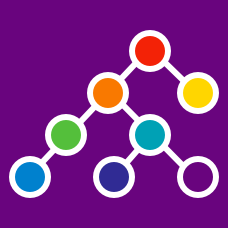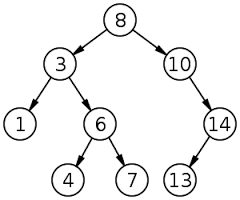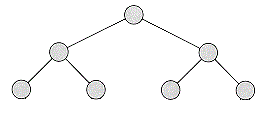Computer Science

# Trees: Level 3 ChallengesFollowing Tree Traversing Techniques can be used to print a Binary Search Tree (BST);

• Preorder traversal (left) (right) root
• Postorder traversal root (left) (right)
• Inorder traversal (left) root (right)

Which of them can be used to print a Binary Search Tree (BST) in Descending Order?

##### this problem is apart of this set

What data structure is required for storing a set of integers such that, deletion of the smallest element and, insertion of an element if not already present in the set, can be done in $O(\log n)$ time?Suppose each number in the list $[ 1,2,3,4,5,6,7 ]$ is assigned to each node in the tree above. How many of the possible 5040 trees are binary search trees?

×